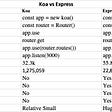# First, let me provide an explanation for the formula known as the Haversine Formula.

Haversine allows you to calculate the distance between two sets of coordinates on a sphere — which is considerably different than calculating distances on a flat plane: Flat Earth folks will be working with the Pythagorean Theorem directly so they can Click Here.

The best explanation I’ve seen personally is taken directly from an ESRI community post by regular contributor SimonKettle¹:

`The Haversine formula is perhaps the first equation to consider when understanding how to calculate distances on a sphere. The word "Haversine" comes from the function:haversine(θ) = sin²(θ/2)The following equation where φ is latitude, λ is longitude, R is earth’s radius (mean radius = 6,371km) is how we translate the above formula to include latitude and longitude coordinates. Note that angles need to be in radians to pass to trig functions:a = sin²(φB — φA/2) + cos φA * cos φB * sin²(λB — λA/2)c = 2 * atan2( √a, √(1−a) )d = R ⋅ c`

The haversine formula is a re-formulation of the spherical law of cosines, but the formulation in terms of haversines is more useful for small angles and distances. — SimonKettle

The author goes on to provide an example in Python as well which I will detail at the base of this

# Javascript — Haversine Formula

Example Usage: https://replit.com/@AbeFlansburg/haversine#index.js

# Ruby — Haversine Formula

Example Usage: https://replit.com/@AbeFlansburg/haversine-1#main.rb

# SQL — Haversine Formula

Keeping in mind that I am not a Master of the SQL and that I was working with a sample data set while using an example seen on StackOverflow²:

`SELECT carrier.code, carrier.description, airport.lat, airport.long, ( 3959 * acos( cos( radians(airport.lat) ) * cos( radians( 61.2181 ) ) * cos( radians(149.9003) - radians(airport.long) ) + sin( radians(airport.lat) ) * sin( radians(61.2181)))) as distance_to_anchorageFROM carrierINNER JOIN airport ON airport.code=carrier.codeWHERE airport.city LIKE '%AK' AND LOWER(carrier.description) LIKE '%inc%'`

where this is the relevant line of code:

`( 3959 * acos( cos( radians(latitude1) ) * cos( radians( latitude2 ) ) * cos( radians(longitude2) - radians(longitude1) ) + sin( radians(latitude1) ) * sin( radians(latitude2))))`

# Python — Haversine

`'''Calculate distance using the Haversine Formula'''def haversine(coord1: object, coord2: object):    import math    # Coordinates in decimal degrees (e.g. 2.89078, 12.79797)    lon1, lat1 = coord1    lon2, lat2 = coord2    R = 6371000  # radius of Earth in meters    phi_1 = math.radians(lat1)    phi_2 = math.radians(lat2)    delta_phi = math.radians(lat2 - lat1)    delta_lambda = math.radians(lon2 - lon1)    a = math.sin(delta_phi / 2.0) ** 2 + math.cos(phi_1) * math.cos(phi_2) * math.sin(delta_lambda / 2.0) ** 2        c = 2 * math.atan2(math.sqrt(a), math.sqrt(1 - a))    meters = R * c  # output distance in meters    km = meters / 1000.0  # output distance in kilometers    meters = round(meters, 3)    km = round(km, 3)    print(f"Distance: {meters} m")    print(f"Distance: {km} km")‍‍‍‍‍‍‍‍‍‍‍‍‍‍‍‍‍‍‍‍‍‍‍‍‍‍‍‍‍‍‍‍‍‍‍‍‍‍‍‍‍‍‍‍‍‍‍‍‍‍‍‍‍‍‍‍‍‍‍‍‍‍‍‍‍‍‍‍‍‍‍‍‍‍‍‍‍‍‍‍‍‍‍‍‍‍‍‍‍‍‍‍‍‍‍‍‍‍‍‍‍‍‍‍‍‍‍‍‍‍‍‍‍‍‍‍‍‍‍‍‍‍‍‍‍‍‍‍‍‍‍‍‍‍‍‍‍‍‍‍‍‍‍‍‍‍‍‍‍‍‍‍‍‍‍‍‍‍‍‍‍‍‍‍‍‍‍‍‍‍‍‍‍‍‍‍‍‍‍‍‍‍‍‍‍‍‍‍‍‍‍‍‍‍‍‍‍‍‍‍‍‍‍‍‍‍‍‍‍‍‍‍‍‍‍‍‍‍‍‍‍‍‍‍‍‍‍‍‍‍‍‍‍‍‍‍‍‍‍‍‍‍‍‍‍‍‍‍‍‍‍‍‍‍‍‍‍‍‍‍‍‍‍‍‍‍‍‍‍‍‍‍‍‍‍‍‍‍‍‍‍‍‍‍‍‍‍‍‍‍‍‍‍‍‍‍‍‍‍‍‍‍‍‍‍‍‍‍‍‍‍`

I hope this can be helpful to someone looking to calculate straight line distances given two sets of latitude and longitude on a sphere.

# Sources

 Kettle, Simon (Oct-5–2017). Distance on a sphere: The Haversine Formula.
https://community.esri.com/t5/coordinate-reference-systems/distance-on-a-sphere-the-haversine-formula/ba-p/902128

 Saadb (Sep-5–2016). SQL query of Haversine formula in SQL server
https://stackoverflow.com/questions/39338167/sql-query-of-haversine-formula-in-sql-server

--

--

--

## More from Abe Flansburg

Devops / Software / Data Engineer / Follower of Jesus Christ

Love podcasts or audiobooks? Learn on the go with our new app.

## Getting Started with ZAPI## Demystifying Python Lambda Functions## Functional Programming: Memoization.## What Would A GUI Terminal Look Like?## Three ways of doing integration tests## Adding authorization to a running Kafka cluster## Abe Flansburg

Devops / Software / Data Engineer / Follower of Jesus Christ

## Express vs Koa, Which Framework is Better?## How to install Node.js and npm using Node Version Manager (NVM)## How to import ESLint issues into SonarQube overview analysis in a frontend application## The Ultimate Node/Docker Setup: Step-by-Step (2022 Update)